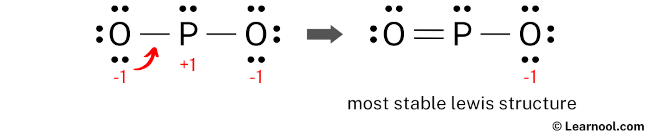# PO2- lewis structure

PO2 has one phosphorus atom and two oxygen atoms.

In PO2 lewis structure, there is one double bond and one single bond around the phosphorus atom, with two oxygen atoms attached to it. The oxygen atom with a double bond has two lone pairs, the oxygen atom with a single bond has three lone pairs, and the phosphorus atom has one lone pair.

Also, there is a negative (-1) charge on the oxygen atom with a single bond.

Contents

## Steps

Use these steps to correctly draw the PO2 lewis structure:

#1 First draw a rough sketch
#2 Mark lone pairs on the atoms
#3 Calculate and mark formal charges on the atoms, if required
#4 Convert lone pairs of the atoms, and minimize formal charges
#5 Repeat step 4 if needed, until all charges are minimized, to get a stable lewis structure

Let’s discuss each step in more detail.

### #1 First draw a rough sketch

• First, determine the total number of valence electrons

In the periodic table, phosphorus lies in group 15, and oxygen lies in group 16.

Hence, phosphorus has five valence electrons and oxygen has six valence electrons.

Since PO2 has one phosphorus atom and two oxygen atoms, so…

Valence electrons of one phosphorus atom = 5 × 1 = 5
Valence electrons of two oxygen atoms = 6 × 2 = 12

Now the PO2 has a negative (-1) charge, so we have to add one more electron.

So the total valence electrons = 5 + 12 + 1 = 18

Learn how to find: Phosphorus valence electrons and Oxygen valence electrons

• Second, find the total electron pairs

We have a total of 18 valence electrons. And when we divide this value by two, we get the value of total electron pairs.

Total electron pairs = total valence electrons ÷ 2

So the total electron pairs = 18 ÷ 2 = 9

• Third, determine the central atom

We have to place the least electronegative atom at the center.

Since phosphorus is less electronegative than oxygen, assume that the central atom is phosphorus.

Therefore, place phosphorus in the center and oxygens on either side.

• And finally, draw the rough sketch

### #2 Mark lone pairs on the atoms

Here, we have a total of 9 electron pairs. And two P — O bonds are already marked. So we have to only mark the remaining seven electron pairs as lone pairs on the sketch.

Also remember that phosphorus is a period 3 element, so it can keep more than 8 electrons in its last shell. And oxygen is a period 2 element, so it can not keep more than 8 electrons in its last shell.

Always start to mark the lone pairs from outside atoms. Here, the outside atoms are oxygens.

So for each oxygen, there are three lone pairs, and for phosphorus, there is one lone pair.

Mark the lone pairs on the sketch as follows:

### #3 Calculate and mark formal charges on the atoms, if required

Use the following formula to calculate the formal charges on atoms:

Formal charge = valence electrons – nonbonding electrons – ½ bonding electrons

For phosphorus atom, formal charge = 5 – 2 – ½ (4) = +1

For each oxygen atom, formal charge = 6 – 6 – ½ (2) = -1

Here, both phosphorus and oxygen atoms have charges, so mark them on the sketch as follows:

The above structure is not a stable lewis structure because both phosphorus and oxygen atoms have charges. Therefore, reduce the charges (as below) by converting lone pairs to bonds.

### #4 Convert lone pairs of the atoms, and minimize formal charges

Convert a lone pair of the oxygen atom to make a new P — O bond with the phosphorus atom as follows:Lone pair of left oxygen is converted, and got the most stable lewis structure of PO2–

In the above structure, you can see that the central atom (phosphorus) forms an octet. Hence, the octet rule is satisfied.

Now there is still a negative (-1) charge on the oxygen atom with a single bond.

This is okay, because the structure with a negative charge on the most electronegative atom is the best lewis structure. And in this case, the most electronegative element is oxygen.

Also, the above structure is more stable than the previous structures. Therefore, this structure is the most stable lewis structure of PO2.

And since the PO2 has a negative (-1) charge, mention that charge on the lewis structure by drawing brackets as follows: